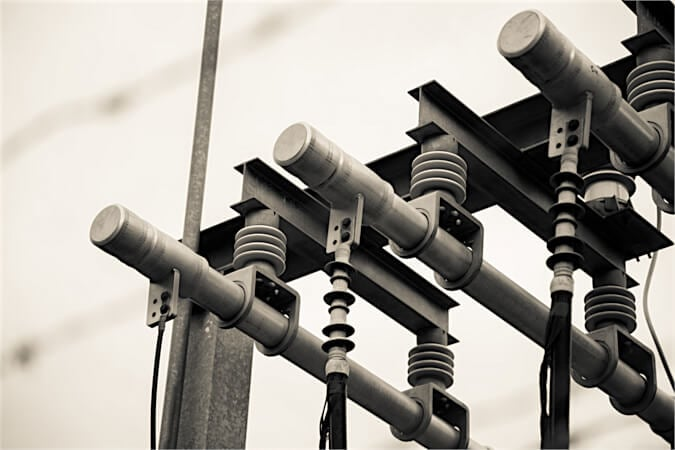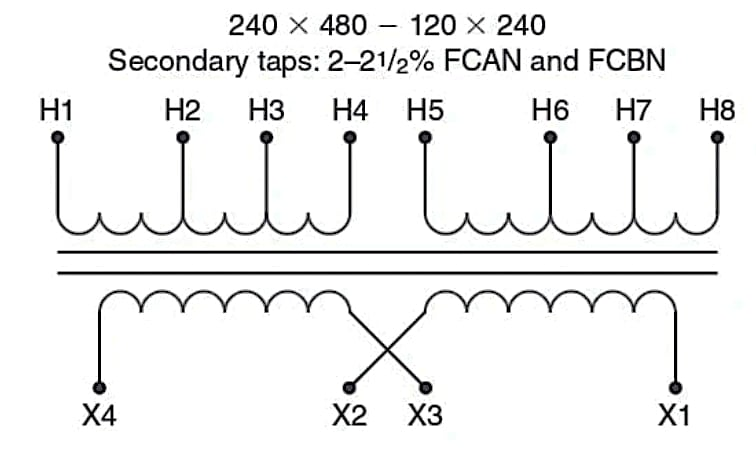Technical Article

# Understanding Transformer Voltage Adjustment Taps

October 11, 2023 by Ahmed Sheikh

## This article explains transformer voltage adjustment taps and their crucial role in maintaining secondary voltage despite fluctuations in the supply.

The voltage supplied to a transformer primary can vary from the nominal value. Most utility companies will provide voltages within 5% of the nominal ratings; however, a voltage drop within a facility may cause the actual voltage supplied to a transformer below the nominal value by more than 5%. The utility supply may also vary. Supplying the transformer primary with a voltage different than the rated primary voltage results in a secondary voltage either higher than or, in most cases, lower than the voltage required by the load. This can cause the load device to operate improperly or not at all. Computers and other electronic devices are especially affected by voltage variation.##### Image used courtesy of Freepik

Transformers are supplied with a way to choose the number of primary turns to compensate for these small changes in voltage to solve this problem.

We know that the number of turns in the transformer winding is proportional to the voltage of the winding. If a transformer had a voltage rating of 480 – 120, there are four times as many turns on the H winding as the X winding. If the actual voltage applied to the H winding were 508 V, then the induced voltage on the X winding would be:

$\frac{508\,Volts}{4}=127\,V$

To make the voltage on the X coil equal 120 V, the ratio of turns of the windings must change. The correct ratio would be:

$\frac{508\,Volts}{120\,Voltage}=4.23\,to\,1$

In other words, the number of turns on the H winding must increase as the applied voltage increases to induce the same voltage on the X winding. The opposite is true if the applied voltage decreases:

$\frac{454\,Volts}{4}=113.5\,V$

The correct ratio is:

$\frac{454\,Volts}{120\,Voltage}=3.78\,to\,1$

To provide rated secondary voltage even with variations in supply voltage, transformers are provided with different numbers of turns on the primary windings that are accessed by use of taps called voltage correction taps. These taps are normally designed to correct voltage variations in 2.5% increments and are referred to as FCAN (full capacity above nominal voltage) and FCBN (full capacity below nominal voltage) taps. The transformer connected with the proper tap will result in the correct secondary voltage at full load amps if the supply voltage is 2.5% above or below nominal voltage values.

Many transformers will have more than one tap for voltages above or below nominal values. For example, a transformer may have 2 2.5% FCAN and 4 2.5% FCBN taps, so the transformer can supply the proper output voltage if the supply voltage is as high as 5% (2  2.5%) above nominal or as low as 10% (4 2.5%) below nominal values. The taps are located at various places along the H winding, so there are more or fewer turns for higher or lower voltages.

### Selecting the Correct Tap

To choose the correct tap, determine the percentage variation of your supply voltage above or below the nominal voltage.

If the supply voltage to a 480 – 240 transformer is 468 V, which tap should be chosen if the transformer has 2 – 2.5% FCAN and 4 – 2.5% FCBN taps?

Find the percentage difference of the supply vs. the nominal voltage:

$\frac{468\,Volts}{480\,Voltage}=97.5\%;the\,difference\,is\,2.5\%,so\,use\,the\,tap\,for\,2.5\%$

#### Tech Tip

When choosing the correct tap, remember the rated voltage of the tap means that the secondary voltage will be at or above the nominal voltage when the supply voltage is equal to or greater than the rated voltage of the tap voltage.

Choose a tap below the calculated value if the supply voltage does not fall exactly on a 2.5% value. For example, If the supply voltage to a 480 – 240 transformer is 437 V, which tap should be chosen if the transformer has 2 – 2.5% FCAN and 4 – 2.5% FCBN taps?

Find the percentage difference of the supply vs. the nominal voltage:

$\frac{437\,Volts}{480\,Voltage}=91\%;the\,difference\,is\,9\%,so\,use\,the\,tap\,for\,10\%(4\times2.5\%)$

The choice of the 10% tap means that if the supply voltage is at least 90% of the nominal voltage of 480 V, the secondary voltage will be at least 240 volts at full transformer load current. This tap will have the least number of turns on the H winding. Figure 1 shows a connection diagram for a dual-winding transformer with 2-2.5% FCAN and FCBN taps. The table shows the value of the voltages 2.5% and 5% above and below nominal voltage and how to make the connections for the voltages.Primary Volt Connect Supply To 504 H4-H5 H1 and H8 492 H3-H5 H1 and H8 480 H3-H6 H1 and H8 468 H2-H6 H1 and H8 456 H2-H7 H1 and H8 252 H1–H5 H4–H8 H1 and H8 240 H1–H6 H3–H8 H1 and H8 228 H1–H7 H2–H8 H1 and H8
##### Figure 1. A connection diagram for a transformer with voltage correction taps. Image used courtesy of Ahmed Sheikh

If the supply voltage does not match the voltage in the table exactly, remember that the listed voltage is the voltage that must be applied to induce the nominal voltage in the secondary. If the supply voltage is less than the listed voltage, choose the voltage tap lower than the supply voltage to ensure the secondary voltage will be equal to or greater than the rated secondary voltage.

For example, if the supply voltage is 462 V and you want a secondary voltage of 240 volts, you must choose the 456 V connection. Selecting the 468 V connection will only provide a rated secondary voltage if the supply voltage is 468 V or higher. If you think of the voltage rating as the number of turns on the H and X windings, the turns ratio for the 468 V connection would be:

$\frac{468\,Volts}{240\,Voltage}=1.95\,to\,1$

If you applied 462 V to this connection, the actual secondary voltage would be:

$\frac{468\,Turns}{240\,Turns}=\frac{462\,Volts}{Sec\,Volts}$

$Sec\,Volts=\frac{462\times240}{468}=237\,V$

This is less than the 240 V rating of the secondary winding. If you chose the 456 V connection, the actual secondary voltage would be:

$\frac{456\,Turns}{240\,Turns}=\frac{462\,Volts}{Sec\,Volts}$

$Sec\,Volts=\frac{462\times240}{456}=243\,V$

### Choosing Voltage Tap Connections

Transformer manufacturers label the tap connections differently, but in all cases, the greater the number of turns, the higher the voltage that can be applied and still result in the nominal secondary voltage. The lower the number of turns, the lower the voltage that can be applied, resulting in the nominal secondary voltage. If the actual supply voltage does not match the voltage of a tap connection exactly, choose the tap connection with a voltage rating lower than the actual supply voltage.

Think about it this way: The voltage tap rating means that if the supply voltage is as great as the tap voltage rating, the transformer's secondary voltage will be as great as the nominal voltage. If the supply voltage is less than the tap voltage rating, the secondary voltage will be less than the nominal rating. We almost always want to see a higher voltage rather than a lower one, so choose the connection that will give the higher voltage. The same rules of transformer voltage correction taps also apply to three-phase transformers.